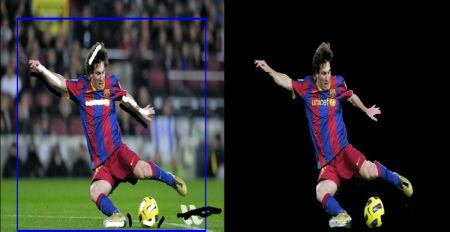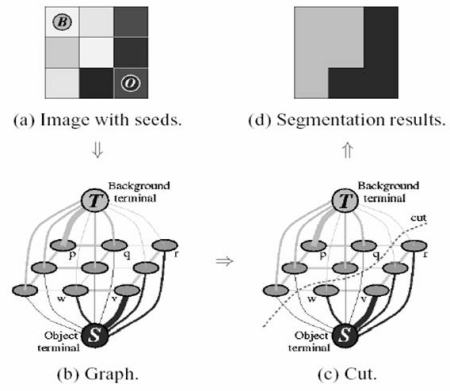# 交互式前景提取使用GrabCut算法¶

### 理论¶### 示例¶

import numpy as np
import cv2 as cv
from matplotlib import pyplot as plt
bgdModel = np.zeros((1,65),np.float64)
fgdModel = np.zeros((1,65),np.float64)
rect = (50,50,450,290)
plt.imshow(img),plt.colorbar(),plt.show()


＃newmask是我手动标记过的mask图像
plt.imshow(img),plt.colorbar(),plt.show()